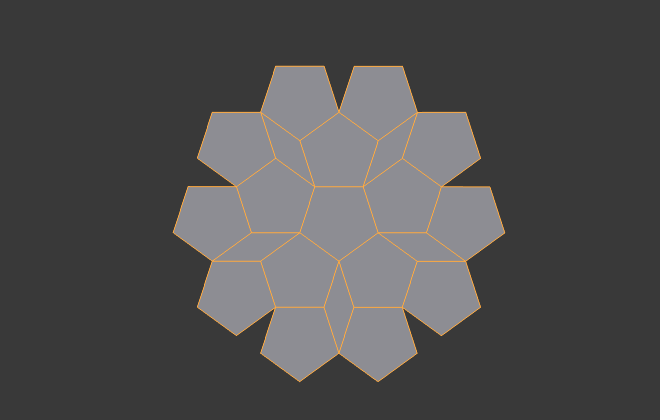# Generative Art - Penrose Tilings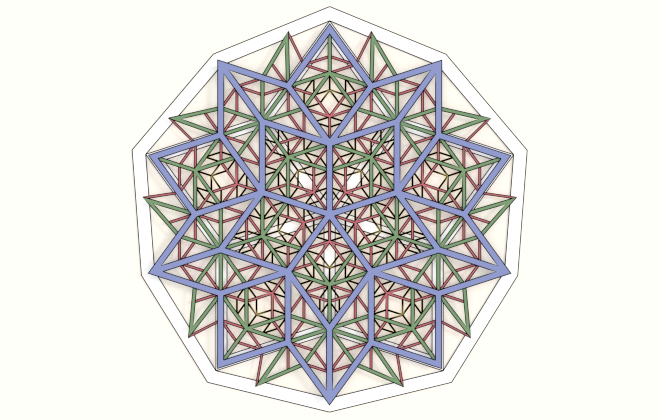Penrose tilings are an interesting group of tilings that have no translational symmetry but are self-similar on different scales. One method to construct them is using substitution, the same method I used for the Pentaflake fractal in the previous post.

There are three types of Penrose tilings:

• P1 has pentagon tiles separated by a five pointed star, a diamond and an odd "boat" or "hat" shape.
• P2 has "kite" and "dart" shaped tiles.
• P3 has two shapes of rhomb or diamond shaped tiles.

All of these tile shapes are based on a pentagon and the golden ratio $$\phi$$.

I'll go through how to construct the tilings in Blender using Sverchok and the Generative Art node.

## P3 Tiling - Rhomb

I'll start at the bottom of the above list with the P3 Penrose tiling made with two types of rhomb or diamond shaped tiles. Each of the diamonds can be divided in half to give two triangles called Robinson triangles.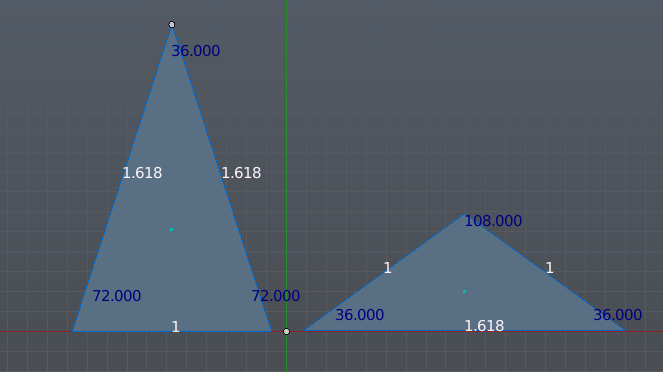The thin triangle on the left is an acute isosceles triangle, and the fat one on the right is an obtuse isosceles triangle. There are three constants that we will use a lot in our eisenxml files used to describe the tilings.

Golden Ratio:

$$\phi = \frac{\sqrt{5}+1}{2}$$

This is the ratio between the long and the short sides for both the thin and fat triangles.
The altitude of each triangle (the distance from the apex to the base) is found from Pythagoras' theorem:

$$x_{thin} = \sqrt{\phi + 3/4}$$
$$x_{fat} = \frac{\sqrt{3-\phi}}{2}$$

Also a useful identity for deriving these formula is:

$$\phi^2 = \phi + 1$$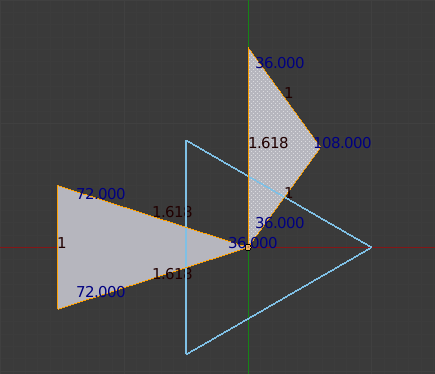Its easy to produce an equilateral triangle in Sverchok using the Circle node with N Vertices set to 3. This give a triangle centered at the origin and pointing to the right, shown as a blue outline above. This following eisenxml scales the equilateral triangle in the x and y directions to give the thin and fat Robinson triangles. It also moves them so one vertex of each triangle is at the origin.

<rules max_depth="100">
<constants phi = "((1+5**0.5)/2)" />
<constants thinx = "({phi}+0.75)**0.5" />
<constants fatx = "0.5*(3-{phi})**0.5" />

<rule name="entry">
<call  rule="thin_tri"/>
<call  rule="fat_tri"/>
</rule>
<rule name="thin_tri">
<instance sx="2*{thinx}/3" sy="1/(3**0.5)" tx="-2*{thinx}/3" shape="tri"/>
</rule>
<rule name="fat_tri">
<instance sx="2*{fatx}/3" sy="({phi}/(3**0.5))" tx="{fatx}/3" ty="{phi}/2" shape="tri"/>
</rule>
</rules>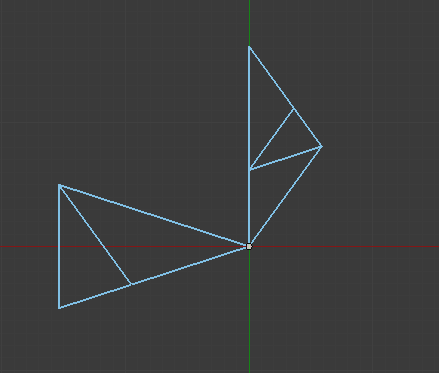Each triangle now needs to be subdivided. The eisenxml below subdivides the thin triangle into one smaller thin and one smaller fat triangle (rule thin_tri_sub). The fat triangle is divided into two smaller fat triangles and one smaller thin triangle (rule fat_tri_sub).

To make the tiling correct we need to mirror (or flip over) two of the smaller copies when we do the substitution. This is achieved by the rx="180" transformation.

The triangles substituted into the thin triangle are scaled by $$s1 = 1 / \phi$$ smaller than the original triangles.

The triangles substituted into the fat triangles are scaled by $$s2 = 1/ \phi^{2}$$.

<rules max_depth="100">
<constants phi = "((1+5**0.5)/2)" />
<constants thinx = "({phi}+0.75)**0.5" />
<constants fatx = "0.5*(3-{phi})**0.5" />
<constants s1 = "1/{phi}" />
<constants s2 = "1/(1+{phi})" />

<rule name="entry">
<call  rule="thin_tri_sub"/>
<call  rule="fat_tri_sub"/>
</rule>

<rule name="thin_tri_sub" max_depth="{md}" successor="thin_tri">
<call tx="-1*{thinx}" ty="0.5" rz="108" sa="{s1}" rule="thin_tri_sub" />
<call rz="108" rx="180" rule="fat_tri_sub" />
</rule>

<rule name="thin_tri">
<instance sx="2*{thinx}/3" sy="1/(3**0.5)" tx="-2*{thinx}/3" shape="tri"/>
</rule>

<rule name="fat_tri_sub" max_depth="{md}" successor="fat_tri">
<call rz="-144"  ty="({phi}-1)" sa="{s2}" rule="thin_tri_sub" />
<call  rz="144" tx="{fatx}" ty="{phi}/2" sa="{s1}" rule="fat_tri_sub" />
<call ty="{phi}" rx="180" sa="{s1}" rule="fat_tri_sub" />
</rule>

<rule name="fat_tri">
<instance sx="2*{fatx}/3" sy="({phi}/(3**0.5))" tx="{fatx}/3" ty="{phi}/2" shape="tri"/>
</rule>

</rules>


To produce a full plane tiling from this we change the entry rule to set 10 thin triangles around the origin, each second triangle also needs to be flipped:

....
<rule name="entry">
<call count="5" rz="72" rule="thin_pair"/>
</rule>

<rule name="thin_pair">
<call  rule="thin_tri_sub"/>
<call rx="180" rz="36" rule="thin_tri_sub"/>
</rule>
....


We could also choose to start with 10 fat triangles instead.

The complete eisenxml and json for the nodes are in the example repo.

The above construction results in all the vertices being doubled. To produce a mesh, use the Apply Matrix and Remove Doubles nodes. Many of the faces will have their normals flipped but this is fixed with the Recalc Normals node.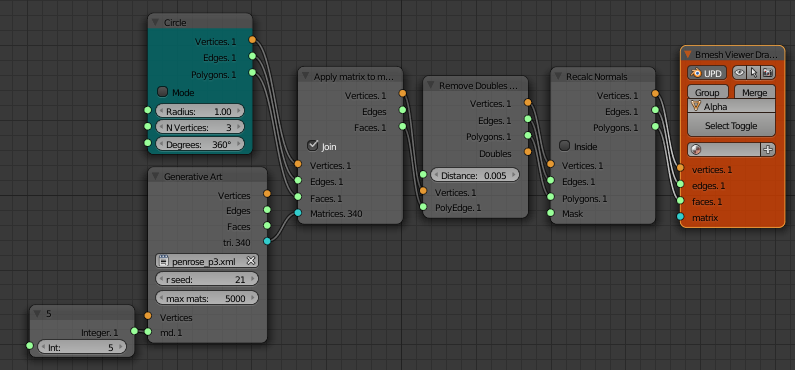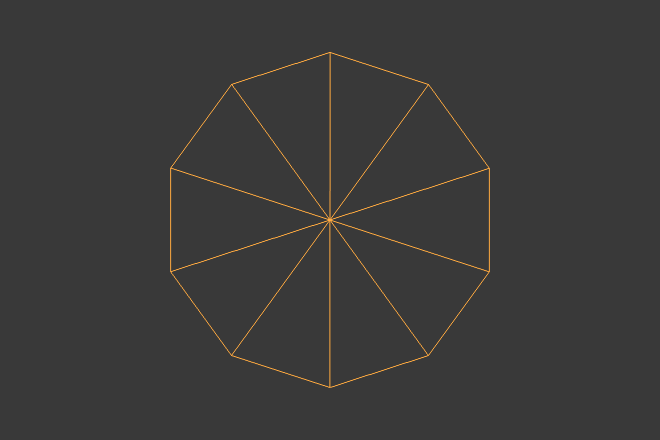At the end of the gif above the Robinson triangle tiling is turned into a proper P3 Rhomb tiling. This is done by selecting one of the longest edges (in Edit mode), then using Select > Select Similar > Length to select all the long edges. Then Mesh > Delete > Dissolve Edges to turn the pairs of fat triangles into fat rhombs. Select and dissolve all the short edges in a similar manner to join the thin triangles into thin rhombs.

## P2 Tiling - Kite and Dart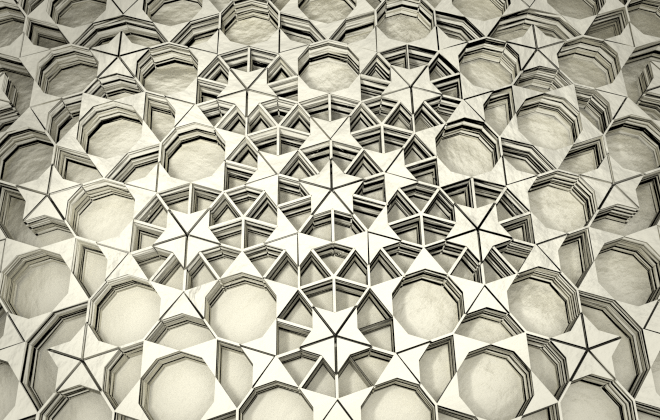The Penrose P2 tiling consists of kite and dart shapes. These kite and dart shapes can also be subdivided into Robinson triangles.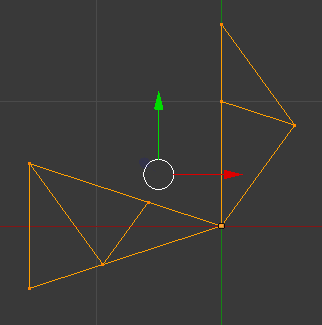This time the subdivide/substitution step involves dividing the thin triangle into two smaller thin triangles and one smaller fat triangle. The fat triangle is divided into one smaller thin and one smaller fat triangle. Notice again that the triangle sides are split in the golden ratio.

...
<rule name="thin_tri_sub" max_depth="{md}" successor="thin_tri">
<call tx="-1*{thinx}" ty="0.5" rz="108" sa="{s1}" rule="thin_tri_sub" />
<call tx="-1*{thinx}" ty="0.5" rx="180" rz="-144" sa="{s1}" rule="thin_tri_sub" />
<call rz="108"  sa="{s1}" rule="fat_tri_sub" />
</rule>

....
<rule name="fat_tri_sub" max_depth="{md}" successor="fat_tri">
<call  rz="-144" ty="{phi}" sa="{s1}" rule="fat_tri_sub" />
<call  rz="108" rx="180" sa="{s1}" rule="thin_tri_sub" />
</rule>
....


full eisenxml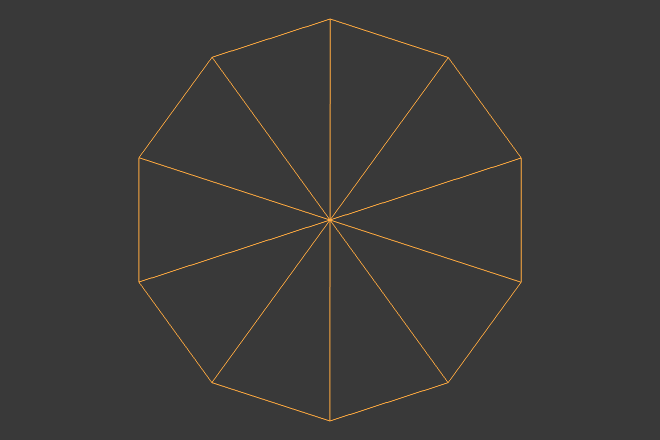Turning the pairs of Robinson triangles into kites and darts is harder for this tiling as both edges within the kites and edges between the kites and darts have the same length. Instead we can use the select similar area tool to select all the thin triangles and give them a different material than the fat triangles.

## P1 Tiling - Pentagonal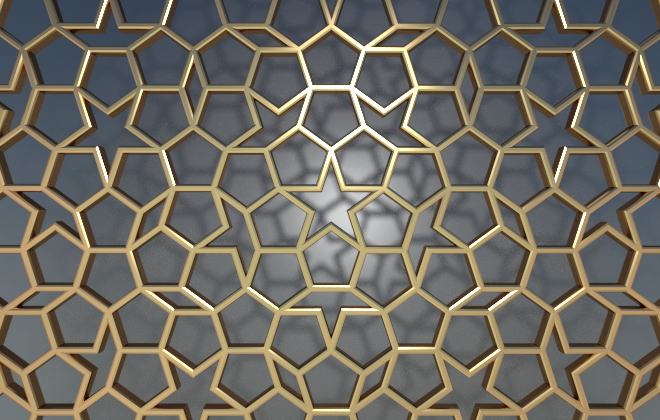The P1 tiling looked much harder to make than the P2 or P3 tilings. The larger number of odd shapes were going to be harder to draw and there are six substitution rules to write.

A closer a look at this diagram on Wikipedia:

showed that the P1 tiling could easily be created from the P3 tiling.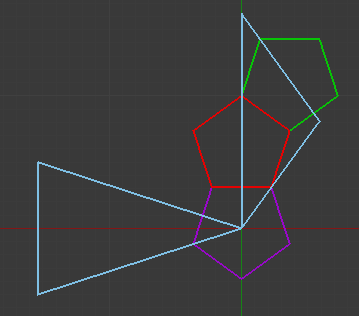Each fat triangle has three pentagons, one centered at a base corner, one centered on a short side and one on a long side.

...
<rule name="fat_tri">
<instance sx="2*{fatx}/3" sy="({phi}/(3**0.5))" tx="{fatx}/3" ty="{phi}/2" shape="tri"/>
<instance ty="{phi}-1" rz="18" sa="{s2}" shape="penta" />
<instance tx="({phi}-1)*{fatx}" ty="{phi}-0.5" rz="-18" sa="{s2}"  shape="penta" />
<instance  rz="-18" sa="{s2}" shape="penta" />
</rule>
...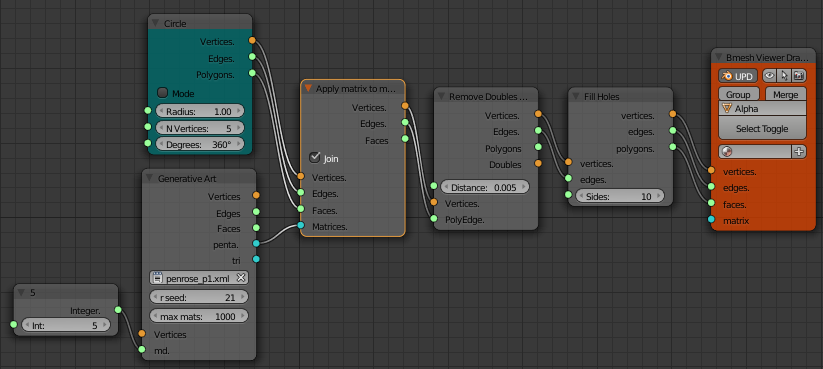This construction doubles up on lots of pentagons but they can be easily removed with the Remove Doubles node. There are also star, diamond and boat shaped gaps between the pentagons. The Sverchok Fill Holes node can be used to fill these.